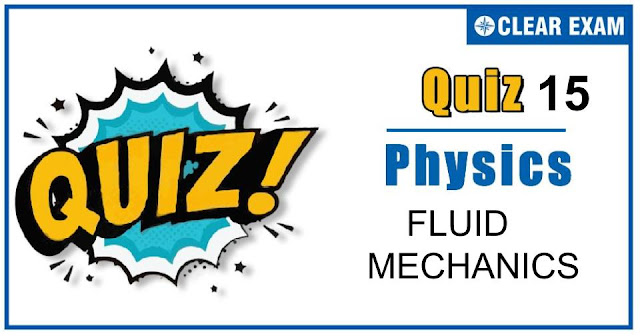## FLUID MECHANICS QUIZ-15

As per analysis for previous years, it has been observed that students preparing for NEET find Physics out of all the sections to be complex to handle and the majority of them are not able to comprehend the reason behind it. This problem arises especially because these aspirants appearing for the examination are more inclined to have a keen interest in Biology due to their medical background. Furthermore, sections such as Physics are dominantly based on theories, laws, numerical in comparison to a section of Biology which is more of fact-based, life sciences, and includes substantial explanations. By using the table given below, you easily and directly access to the topics and respective links of MCQs. Moreover, to make learning smooth and efficient, all the questions come with their supportive solutions to make utilization of time even more productive. Students will be covered for all their studies as the topics are available from basics to even the most advanced. .

Q1. Water falls from a tap , down the streamline
•  Area decreases
•  Area increases
•  Velocity remains same
•  Area remains same
Solution
Area decreases

Q2.A vessel of area of cross-section A has liquid to a height H. There is a hole at the bottom of vessel having area of cross-section a. The time taken to decrease the level from H1 to H2 will be
•  A/a √(2/g)[√(H1 )-√(H2 )]
•  √2gh
•  √(2gh(H1-H2))
•  A/a √(g/2)[√(H1 )-√(H2 )]
Solution
A/a √(2/g)[√(H1 )-√(H2 )]

Q3.  The total weight of a piece of wood is 6 kg. In the floating state in water its 1/3 part remains inside the water. On this floating solid, what maximum weight is to be put such that the whole of the piece of wood is to be drowned in the water?>
•   12kg
•  10kg
•  14kg
•  15kg
Solution
Given, 6g=V/3×〖10〗3×g …(i) And (6+m)g=V×〖10〗3×g …(ii) Dividing Eq.(ii) by Eq. (ii) by Eq. (i), we get (6+m)/6=3 Or m=18-6=12 kg

Q4. A soap bubble in air (two surface) has surface tension 0.03Nm(-1). Find the gauge pressure inside a bubble of diameter 30 mm.
•  2pa
•  4pa
•  16pa
•  8pa
Solution
Gauge pressure=4T/R=(4×0.03)/(30/20×〖10〗(-3) )=8 Pa

Q5.A capillary tube of radius R and length L is connected in series with another tube of radius R/2 and lengthL/4. If the pressure difference across the two tubes taken together is p, then the ratio of pressure difference across the first tube to that across the second tube is
•  1:4
•  1:1
•  4:1
•  1:1
Solution
Volume of liquid flowing per second through each of the two tubes in series will be the same. So V=(π p1 R4)/(8 η L)=(πp2 (R/2)4)/(8η(L/2)) or p1/p2 =1/4

Q6. A body floats in water with one-third of its volume above the surface of water. If it is placed in oil, it floats with half of its volume above the surface of the oil. The specific gravity of the oil is
•  5/3
•  4/3
• 3/2
•  1
Solution
Weight of body = weight of water displaced = weight of oil displaced ⇒2/3 Vρw g=1/2 Vρ0 g ⇒ρ0=4/3 ρw ∴ Specific gravity of oil =ρ0w =4/3

Q7.The excess pressure inside one soap bubble is three times that inside a second soap bubble, then the ratio of their surface areas is
•  1:9
•  1:3
•  3:1
•  1:27
Solution
Accordingly 4T/r1 =3×4T/r2 ⟹ r1/r2 =1/3 Ratio of surface areas A1/A2>(〖4πr〗12)/(〖4πr〗22 )=1/9

Q8.A streamline body with relative density ρ1 falls into air from a height h1 on the surface of a liquid of relative densityρ2, wherep2>p1. The time of immersion of the body into the liquid will be
•  √(2 h1/g)
•  T√(2 h1/g)×ρ12
•  √((2h1)/g)×ρ12
•  √((2h1)/g)×ρ121))
Solution
If V is the volume of the body, its weight=V ρ_1 g. Velocity gained by body when it falls from a height h_1=√(2gh_1 ) The weight of liquid displaced by the body as body starts immersing into the liquid =V ρ_2 g. The net retarding force on the body when it starts going in the liquid F=V(ρ_2-ρ_1)g ∴ Retarding, a=F/(Vρ1 )=[V(ρ21 )g/(V ρ1 )] The time of immersion of the body is that time in which the velocity of the body becomes zero. Using the relation v=u+at, we have v=0,u=√(2gh_1 ), a=-V(ρ21 )g/(V ρ1 )=-((ρ21)/ρ1 )g; We have =0=√(2gh1 )-((ρ21)/ρ1 )gt Or t=√((2h1)/g)×(ρ1/(ρ21 ))

Q9.A liquid does not wet the sides of a solid, if the angle of contact is
•  Obtuse
•  90°
•  acute
•  zero
Solution
The liquid which do not wet the solid have obtuse angle of contact. For mercury and glass, the angle of contact is 135°.

Q10. A log of wood of mass 120 kg floats in water. The weight that can be put on raft to make it just sink, should be (density of wood =600 kg/m3)
•  80kg
•  50kg
•  60kg
• 30kg
Solution
Volume of log of wood V=mass/density=120/600=0.2m3 Let x weight that can be put on the log of wood So weight of the body =(120+x)×10 N Weight of displaced liquid =Vσg=0.2×〖10〗3×10 N The body will just sink in liquid if the weight of the body will be equal to the weight of displaced liquid ∴(120+x)×10=0.2×〖10〗3×10 ⇒120+x=200 ∴x=80 kg## Want to know more

Please fill in the details below:

## Latest NEET Articles\$type=three\$c=3\$author=hide\$comment=hide\$rm=hide\$date=hide\$snippet=hide

Name

ltr
item
BEST NEET COACHING CENTER | BEST IIT JEE COACHING INSTITUTE | BEST NEET, IIT JEE COACHING INSTITUTE: FLUID MECHANICS QUIZ-15
FLUID MECHANICS QUIZ-15